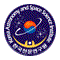# KVN and VERA Array (KaVA)

## Sensitivity

When a target source is observed, a noise levelfor each baseline can be expressed as(3)

where k is Boltzmann constant,is quantization efficiency (~ 0.88), Tsys is system noise temperature, SEFD is system equivalent flux density, Ae is antenna effective aperture area (in whichandare the aperture efficiency and antenna diameter, respectively), B is the bandwidth, andis on-source integration time. Note that for an integration time beyond 3 minutes (in the K band), the noise level expected by equation (3) cannot be attained because of the coherence loss due to the atmospheric fluctuation. Thus, for finding fringe within a coherence time, the integration timecannot be longer than 3 minutes. For VLBI observations, signal-to-noise ratio (S/N) of at least 5 and usually 7 is generally required for finding fringes.

A resultant image noise levelcan be expressed as(4)

If the array consists of identical antennas, an image noise levels can be expressed as(5)

whereis the number of antennas. Using the typical antenna parameters (Table 10), baseline and image sensitivity values of KaVA are calculated as listed in Table 11.
Table 10 : Parameters of each antenna.
KVN VERA
Band Tsys[K]SEFD [Jy] Tsys[K]SEFD [Jy]
K 100 0.6 1328 120 0.5 2343
Q 150 0.6 1992 250 0.5 4881
Table 11:Sensitivity of each array.
Baseline sensitivity [mJy] Image sensitivity [mJy]
Band VERA-VERA KVN-KVN VERA-KVN VERA KVN KaVA
K 10.7 6.1 8.1 0.4 0.3 0.2
Q 22.4 9.1 14.3 0.8 0.5 0.3
Sensitivities (1) are listed in unit of mJy.
Integration time of 120 seconds and 4 hours are assumed for baseline and image
sensitivities, respectively. Total bandwidth of 256 MHz (for continuum emission)
is assumed for all the calculations. In the case of narrower bandwidth of 15.625 KHz
(for maser emission), sensitivities can be calculated by multiplying a factor of 128.

Figures 9 and Figures 10 show the system noise temperature at Mizusawa and Ulsan, respectively. For Mizusawa, receiver noise temperatures are also plotted.

Note that the receiver temperature of the VERA antenna includes the temperature increase due to the feedome loss and the spill-over effect. In Mizusawa, typical system temperature in the K band isK in fine weather of winter season, but sometimes rises aboveK in summer season. The system temperature at Iriki station shows a similar tendency to that in Mizusawa. In Ogasawara and Ishigakijima, typical system temperature is similar to that for summer in Mizusawa site, with typical optical depth of. The typical system temperature in the Q band in Mizusawa isK in fine weather of winter season, andK in summer season. The typical system temperature in Ogasawara and Ishigakijima in the Q band is larger than that in Mizusawa also.

The typical system temperature in the K band at all KVN stations is around 100 K in winter season. In summer season, it increases up to300 K. In the Q band, the typical system temperature is around 150 K in winter season and 250 K in summer season at Yonsei and Tamna. The system temperature of Ulsan in the Q band is about 40 K lower than the other two KVN stations. This is mainly due to the difference in receiver noise temperature (see Table 5).KaVA ( KVN and VERA Array )